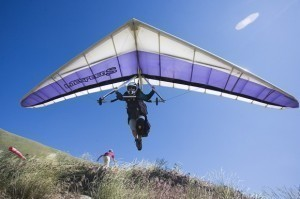# Dimensions of a Hang Glider

Since its emergence, hang gliding has become avery popular sport. There are now numerous models available. Some of them are listed below.

### Dimensions of a Hang Glider: Amour 139 and 159

The Amour 139 hang glider has a wing area of 12.5 m2 and wing span of 8.85 m. The aspect ratio is 6.3 and the minimum pilot weight is 48 kg. The maximum pilot weight is 80 kg. The nose angle is 121 degrees. The Amour 159 has a wing area of 14.6 m2 and wing span of 9.85 m.

The aspect ratio is 6.7 and the hang glider weight is 29 kg. The minimum pilot weight is 64 kg and the max pilot weight is 93 kg. The minimum speed is 24 km/h and the maximum speed is 93 km/h. The max glide ratio is 11.5 L/H. The packed length is 5.9 m.

### Dimensions of a Hang Glider: Breeze and Ace

The wing area of the Breeze hang glider is 15 m2 and the wing span is 9.4 m. The aspect ratio is 5.6 and the hang glider weight is 27 kg. The minimum pilot weight is 65 kg. The maximum pilot weight is 80 kg.

The minimum speed is 25 km/h and the maximum speed is 80 km/h. The maximum glide ratio is 10 L/H. The maximum glide ratio speed is 50 km/h. The nose angle is 15 degrees.

The Ace 150 has a 13.9 m2 wing area and 9.6 wing span. The packed length short is 4.2 m. The battens are 32 and the angle is 122 degrees.

### Dimensions of a Hang Glider: ASG 21

The ASG 21 AA has a 12.1 m2 wing area and 9.4 wing span. The aspect ratio is 6.4. The hang glider weight is 20 kg. The minimum pilot weight is 50 kg and the maximum pilot weight is 61 kg. The packed length is 4.88 m. The ASG 21A has a wing area of 13.5 m2 and a wing span of 8.8 m.

The aspect ratio is 6.4 and the hang glider weight is 20 kg. The minimum pilot weight is 59 kg and the maximum pilot weight is 70 kg. The packed length of the ASG 21A hang glider is 5.18 m and the nose angle is 118 degrees.

### Dimensions of a Hang Glider: Archaeopteryx

The Archaeopteryx was made in 1998 and is designed only for advanced pilots. The wing area is 14 m2 and the wing span is 13 m. The aspect ratio is 12.07 and the hang glider weight is 45 kg.

The minimum pilot weight is 7 kg and the maximum pilot weight is 110 kg. The minimum speed is 29 km/h and maximum speed is 140 km/h. The maximum glide ratio of the Archaeopteryx is 24 L/H. The minimum sink rate is 0.6 m/s. The packed length is 6 m.

The dimensions of a hang glider as specified here are a demonstration of the different varieties you will come across. Manufacturers are continuously coming up with new designs to entice aficionados.